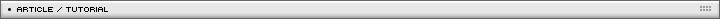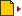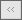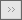Drawing a Mandelbrot set Page 1/1[ July 06, 2006 ] Milan Toth (MilGra) Fractals in Actionscript 3! Milgra guides you through the math behind a the famous Mandelbrot set and shows how fast is AS 3

Drawing a Mandelbrot set

Let's create a Mandelbrot set drawing algorythm. Mandelbrot set marks the set of points in the complex plane where z(i) = z(i-1)*z(i-1) + c function does not tend to infinity. These points marked black, but the nearby points, which aren't in the set, is colored according to how fast they tend to infinity. So we can draw very spectacular nutmeats :) And we can zoom in continously.

Complex numbers have two parts: a real and an imaginery part: z = ( r , i )
Addition: z1 + z2 = ( r1 + r2 , i1 + i2 )
Multiplication: z1z2 = ( r1r2 - i1i2 , r1i2 + r2i1 ).

Knowing this generating the set is childgame :)

We are lucky, because AS3s virtual machine is really fast, so we don't have to use timing like we did it in AS2-

Create a new project named MandelbrotSet, and type:

```//begin
//
//
package
{
//
//
//
import flash.display.Sprite;
import flash.display.Bitmap;
import flash.display.BitmapData;
import flash.display.StageScaleMode;
//
//
//
public class MandelbrotSet extends Sprite
{
//
//
//
private var mbCNT:Bitmap;
private var mbBMP:BitmapData;
//
private var maxCycles:int = 128; // how many cycles should we check
//
private var LEFT:Number = -2; // real axis minimum
private var RIGHT:Number = 1; // real axis maximum
private var BOTTOM:Number = -1; //imaginary axis minumum
private var TOP:Number = 1; // imaginary axis maximum
//
private var WTH:int = 550; //canvas width
private var HTH:int = 380; //canvas height
//
private var XSTEPPING:Number = ( RIGHT - LEFT ) / WTH;
private var YSTEPPING:Number = ( TOP - BOTTOM ) / HTH;
//
//
//
private var zr:Number;
private var zi:Number;
private var cr:Number;
private var ci:Number;
private var zrsq:Number;
private var zisq:Number;```

We defined constants, and working variables. We should use int instead of Number where it is possible, because the cpu doesn't have to do floating point calculations, so it is much faster. We need a BitmapData, which will be contained by a Bitmap instance.

```		//
//
//
public function MandelbrotSet( )
{
//
stage.scaleMode = StageScaleMode.NO_SCALE;
//
mbBMP = new BitmapData( WTH , HTH , false , 0x000000 );
mbCNT = new Bitmap( mbBMP );
//
// much faster with for than do while
//
for ( var a:int = 0 ; a < WTH ; ++a )

for ( var b:int = 0 ; b < HTH ; ++b )
calcPoint( a , b );
//
}```

In the constructor we create our Bitmap, and for every point of our canvas we calculate the color of the related point in the complex plane.

```		//
//
//
private function calcPoint( xp:int , yp:int ):void
{
//
zr = 0;
zi = 0; //
cr = LEFT + XSTEPPING * xp;
ci = BOTTOM + YSTEPPING * yp;
//
zrsq = 0;
zisq = 0;
//
for ( var a:int = 0 ; a < maxCycles ; ++a )

{
//
zi = zr * zi * 2 + ci;
zr = zrsq - zisq + cr;
//
zrsq = zr * zr;
zisq = zi * zi;
//
if ( zrsq + zisq > 4 ) //
{

//
var color:Number = a << 16 | ( a + 50 ) << 8 | a ;
mbBMP.setPixel( xp , yp , color );
return;
//
}

//
}
//
mbBMP.setPixel( xp , yp , 0x000000 );
//
}
//
//
//
}

//
//
//
}
//
//
//end```

Rendering is made by this function, if the absolute value of the recursively generated complex number ( which is the diagonal of the real and imaginary side-length triangle ) is bigger than 2, then it leaves the origin-centered circle with 2 radius, so it tends to infinity.

Two questions may appear: where does zi = zr*zi*2 + ci comes? Simple: we have to use the two best friends of the programmer: pencil and paper, and work on the a z(i) = z(i-1)z(i-1) + c equality, like this:

z(2) = z(1)*z(1) + c
(zr2,zi2) = (zr1,zi1)(zr1,zi1) + (cr,ci);
(zr2,zi2) = ( zr1*zr1 - zi1*zi1 , zr1*zi1 + zr1*zi1 ) + ( cr , ci );
zr2 = zr1*zr1 - zi1*zi1 + cr;
zi2 = zr1*zi1 + zr1*zi1 + ci = 2*zr1*zi1 + ci;
|zi| > 2 -> we have to stay in the circle with the radius 2
|zi| = sqrt( zr*zr + zi*zi ) > 2;

The other question: what the hell is this line:

`a << 16 | ( a + 50 ) << 8 | a ;`

Simple, we needed a 24 bit length colour code from a number under 128. So, i shifted 128 with 16 bits, to the "red" range ( 2(25) - 2(16) ), then i added 50, because i wanted green to be the dominant color, then shifted left with 8 bits to the green range ( 2(15) - 2(8) ), and i left it in the "blue" range, and made a logical "OR" between these values.

If you have flash player 9, you can check the set here.
You can compare it with the AS2 version here.

This is quite good, but let's make it more spectacular. AS3 virtual machine is fast enough to make a real time Mandelbrot rotozoom. We have to shrink the are represented by LEFT, RIGHT, TOP, BOTTOM constants, and rotate every point before the calculation. Let's see:

```//begin
//
//
package
{
//
//
//
import flash.display.Sprite;
import flash.display.Bitmap;
import flash.display.BitmapData;
import flash.display.StageScaleMode;
import flash.utils.*;
import flash.events.TimerEvent;
import flash.geom.Point;
//
//
//
public class MandelbrotSet extends Sprite
{

//
//
//
private var mbCNT:Bitmap;
private var mbBMP:BitmapData;
private var moveTimer:Timer;
//
private var maxCycles:int = 32;
private var planeRot:Number = 0;
//
private var XCENTER:Number = -1.5;
private var YCENTER:Number = 0;
//
private var LEFT:Number = -2;
private var RIGHT:Number = 1;
private var TOP:Number = 1;
private var BOTTOM:Number = -1;
//
private var WTH:int = 200;
private var HTH:int = 200;
//
private var XSTEPPING:Number;
private var YSTEPPING:Number;
//
private var zr:Number;
private var zi:Number;
private var cr:Number;
private var ci:Number;
private var zrsq:Number;
private var zisq:Number;
//
//
//
public function MandelbrotSet( )

{
//
stage.scaleMode = StageScaleMode.NO_SCALE;
//
mbBMP = new BitmapData( WTH , HTH , false , 0x110000 );
mbCNT = new Bitmap( mbBMP );
//
moveTimer = new Timer( 10 );
moveTimer.start( );
//
}

//
//
//
private function zoom ( eventOBJ:TimerEvent ):void
{
//
LEFT += ( XCENTER - LEFT )/32;
RIGHT += ( XCENTER - RIGHT )/32;
BOTTOM += ( YCENTER - BOTTOM )/32;
TOP += ( YCENTER - TOP )/32;
//
XSTEPPING = ( RIGHT - LEFT ) / WTH;
YSTEPPING = ( TOP - BOTTOM ) / HTH;
//
planeRot += Math.PI/32;
//
for ( var a:int = 0 ; a < WTH ; ++a )

for ( var b:int = 0 ; b < HTH ; ++b )
calcPoint( a , b );
//
}

//
//
//
private function calcPoint( xp:int , yp:int ):void
{
//
var length:Number = Math.sqrt( xp*xp + yp*yp );
var angle:Number = Math.atan2( yp , xp ) + planeRot;
var newPos:Point = Point.polar( length , angle );
//
zr = 0;
zi = 0;
cr = LEFT + XSTEPPING * newPos.x;
ci = BOTTOM + YSTEPPING * newPos.y;
//
zrsq = zr*zr;
zisq = zi*zi;
//
for ( var a:int = 0 ; a < maxCycles ; ++a )

{
//
zi = zr * zi * 2 + ci;
zr = zrsq - zisq + cr;
//
zrsq = zr * zr;
zisq = zi * zi;
//
if ( zrsq + zisq > 4{
//
var color:int = a*4 << 16 | ( a*4 + 50 ) << 8 | a*4 ;
mbBMP.setPixel( xp , yp , color );
return;
//
}

//
}
//
mbBMP.setPixel( xp , yp , 0x000000 );
//
}
//
//
//
}

//
//
//
}
//
//
//end```

Watch it here.

Beautyful, beautyful. Like a 4K intro written in ASM for Assembly 94' .
Those were the beautyful days, but they are returning :)Name: Milan Toth (MilGra) Location: Koka, Hungary Age: 24 Flash experience: 4 years Job: flash developer/web designer Website: http://www.tetrapod.ini.hu/| Homepage | News | Games | Articles | Multiplayer Central | Reviews | Spotlight | Forums | Info | Links | Contact us | Advertise | Credits || www.smartfoxserver.com | www.gotoandplay.biz | www.openspace-engine.com | gotoAndPlay() v 3.0.0 -- (c)2003-2008 gotoAndPlay() Team -- P.IVA 03121770048